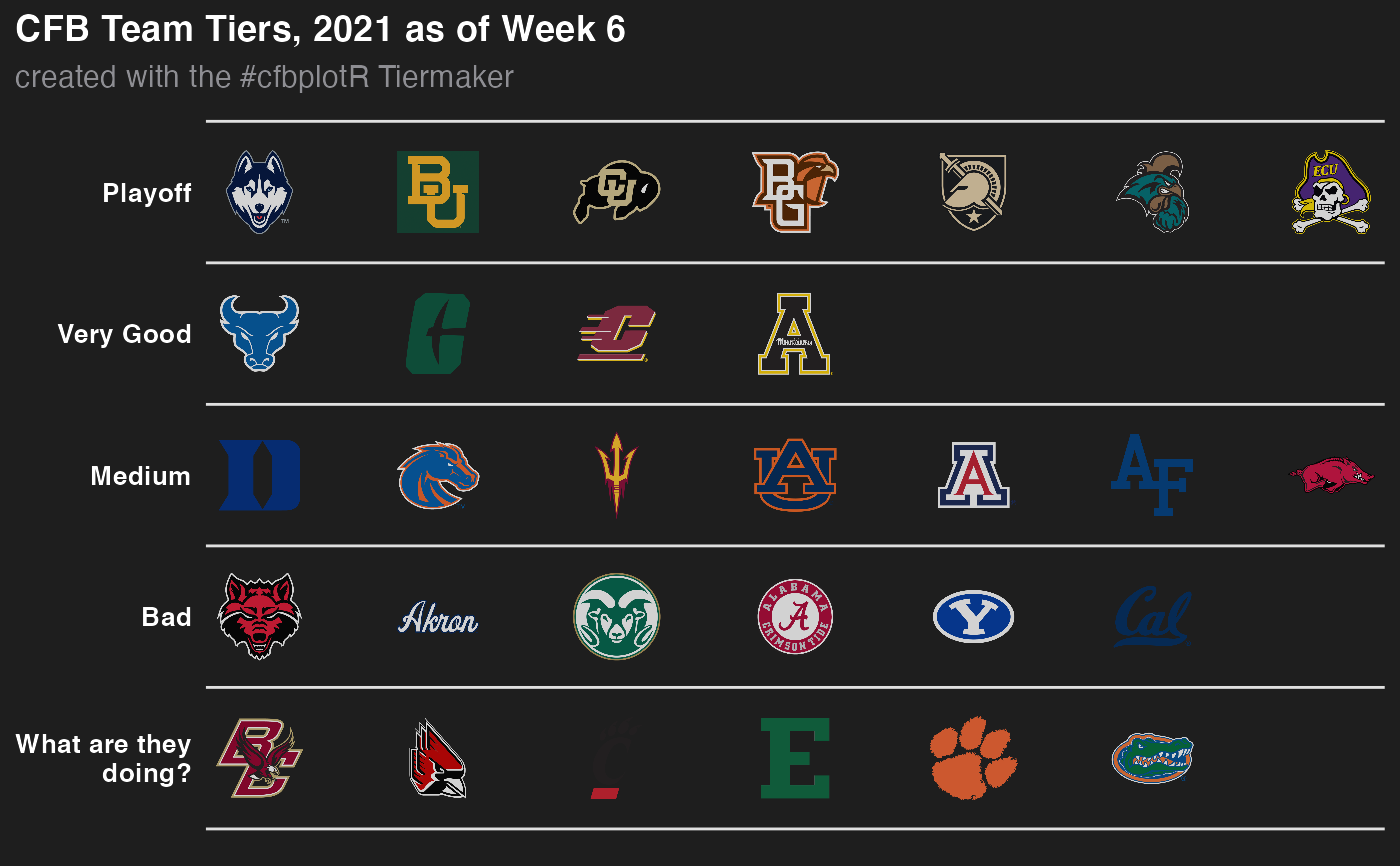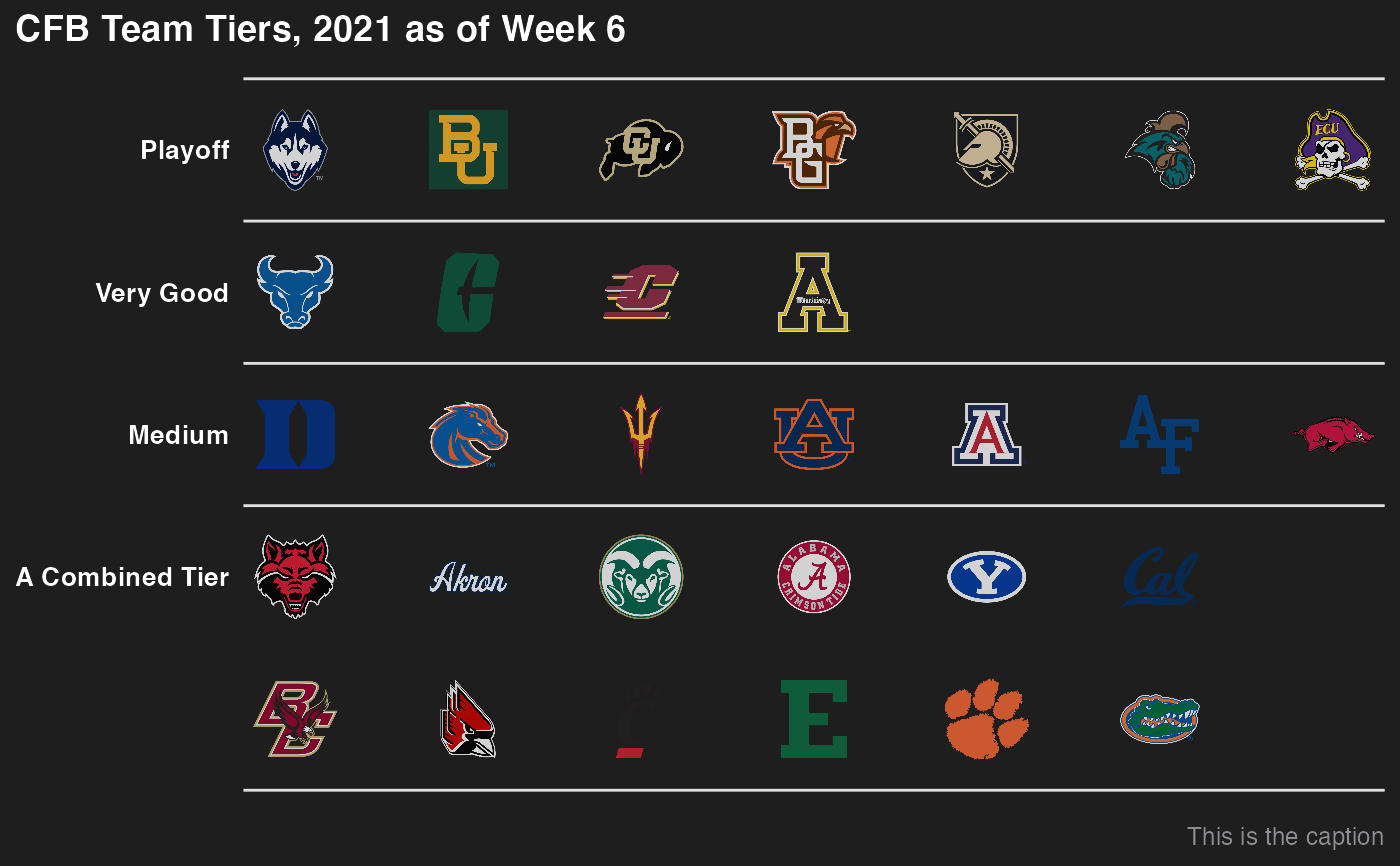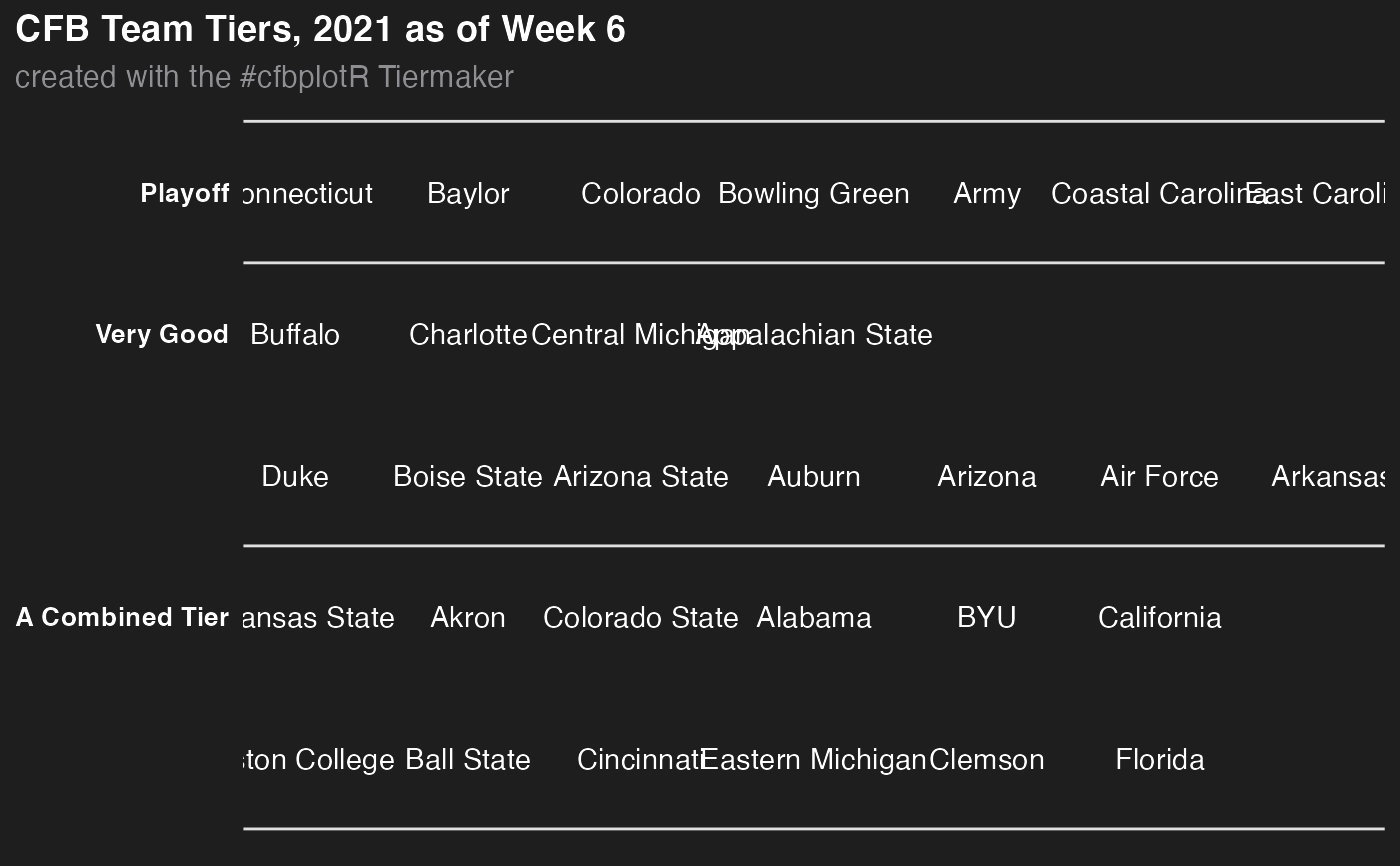This function sets up a ggplot to visualize CFB team tiers.

cfb_team_tiers(
data,
title = "CFB Team Tiers, 2021 as of Week 6",
subtitle = "created with the #cfbplotR Tiermaker",
caption = NULL,
tier_desc = c(1 = "Playoff", 2 = "Very Good", 3 = "Medium", 4 = "Bad", 5 =
"What are they doing?", 6 = "", 7 = ""),
presort = FALSE,
alpha = 0.8,
width = 0.075,
no_line_below_tier = NULL,
devel = FALSE
)

## Arguments

data A data frame that has to include the variables tier_no (the number of the tier starting from the top tier no. 1) and team (the team name). team should be one of valid_team_names(). If data includes the variable tier_rank, these ranks will be used within each tier. Otherwise, if presort = FALSE, the function will assume that data is already sorted and if presort = TRUE, teams will be sorted alphabetically within tiers. The title of the plot. If NULL, it will be omitted. The subtitle of the plot. If NULL, it will be omitted. The caption of the plot. If NULL, it will be omitted. A named vector consisting of the tier descriptions. The names must equal the tier numbers from tier_no If FALSE (the default) the function assumes that the teams are already sorted within the tiers. Will otherwise sort alphabetically. The alpha channel of the logos, i.e. transparency level, as a numerical value between 0 and 1. The desired width of the logo in npc (Normalised Parent Coordinates). Vector of tier numbers. The function won't draw tier separation lines below these tiers. This is intended to be used for tiers that shall be combined (see examples). Determines if logos shall be rendered. If FALSE (the default), logos will be rendered on each run. If TRUE the team names will be plotted instead of the logos. This is much faster and helps with the plot development.

## Examples

# \donttest{
library(ggplot2)
library(dplyr, warn.conflicts = FALSE)
team_names <- valid_team_names("FBS")
# remove conference logos from this example
team_names <- team_names[1:30]

# Build the team tiers data frame
# This is completely random!
df <- data.frame(
tier_no = sample(1:5, length(team_names), replace = TRUE),
team = team_names
) %>%
dplyr::group_by(tier_no) %>%
dplyr::mutate(tier_rank = sample(1:n(), n()))

# Plot team tiers
cfb_team_tiers(df)# Create a combined tier which is useful for tiers with lots of teams that
# should be split up in two or more rows. This is done by setting an empty
# string for the tier 5 description and removing the tier separation line
# below tier number 4.
# This example also shows how to turn off the subtitle and add a caption
cfb_team_tiers(df,
subtitle = NULL,
caption = "This is the caption",
tier_desc = c("1" = "Playoff",
"2" = "Very Good",
"3" = "Medium",
"4" = "A Combined Tier",
"5" = ""),
no_line_below_tier = 4)# For the development of the tiers, it can be useful to turn off logo image
# rendering as this can take quite a long time. By setting devel = TRUE, the
# logo images are replaced by team abbreviations which is much faster
cfb_team_tiers(df,
tier_desc = c("1" = "Playoff",
"2" = "Very Good",
"3" = "",
"4" = "A Combined Tier",
"5" = ""),
no_line_below_tier = c(2, 4),
devel = TRUE)# }